Professor Murray

Parametric Curves

Slide Duration:

Integration by Parts

24m 52s

Intro
0:00
Important Equation
0:07
Where It Comes From (Product Rule)
0:20
Why Use It?
0:35
Lecture Example 1
1:24
Lecture Example 2
3:30
Shortcut: Tabular Integration
7:34
Example
7:52
Lecture Example 3
10:00
Mnemonic: LIATE
14:44
Ln, Inverse, Algebra, Trigonometry, e
15:38
-1
-2
Integration of Trigonometric Functions

25m 30s

Intro
0:00
Important Equation
0:07
Powers (Odd and Even)
0:19
What To Do
1:03
Lecture Example 1
1:37
Lecture Example 2
3:12
Half-Angle Formulas
6:16
Both Powers Even
6:31
Lecture Example 3
7:06
Lecture Example 4
10:59
-1
-2
Trigonometric Substitutions

30m 9s

Intro
0:00
Important Equations
0:06
How They Work
0:35
Example
1:45
Remember: du and dx
2:50
Lecture Example 1
3:43
Lecture Example 2
10:01
Lecture Example 3
12:04
-1
-2
Partial Fractions

41m 22s

Intro
0:00
Overview
0:07
Why Use It?
0:18
Lecture Example 1
1:21
Lecture Example 2
6:52
Lecture Example 3
13:28
-1
-2
Integration Tables

20m

Intro
0:00
Using Tables
0:09
Match Exactly
0:32
Lecture Example 1
1:16
Lecture Example 2
5:28
Lecture Example 3
8:51
-1
-2
Trapezoidal Rule, Midpoint Rule, Left/Right Endpoint Rule

22m 36s

Intro
0:00
Trapezoidal Rule
0:13
Graphical Representation
0:20
How They Work
1:08
Formula
1:47
Why a Trapezoid?
2:53
Lecture Example 1
5:10
Midpoint Rule
8:23
Why Midpoints?
8:56
Formula
9:37
Lecture Example 2
11:22
Left/Right Endpoint Rule
13:54
Left Endpoint
14:08
Right Endpoint
14:39
Lecture Example 3
15:32
-1
-2
Simpson's Rule

21m 8s

Intro
0:00
Important Equation
0:03
Estimating Area
0:28
Difference from Previous Methods
0:50
General Principle
1:09
Lecture Example 1
3:49
Lecture Example 2
6:32
Lecture Example 3
9:07
-1
-2
Improper Integration

44m 18s

Intro
0:00
Horizontal and Vertical Asymptotes
0:04
Example: Horizontal
0:16
Formal Notation
0:37
Example: Vertical
1:58
Formal Notation
2:29
Lecture Example 1
5:01
Lecture Example 2
7:41
Lecture Example 3
11:32
Lecture Example 4
15:49
Formulas to Remember
18:26
Improper Integrals
18:36
Lecture Example 5
21:34
Lecture Example 6 (Hidden Discontinuities)
26:51
-1
-2
Section 2: Applications of Integrals, part 2
Arclength

23m 20s

Intro
0:00
Important Equation
0:04
Why It Works
0:49
Common Mistake
1:21
Lecture Example 1
2:14
Lecture Example 2
6:26
Lecture Example 3
10:49
-1
-2
Surface Area of Revolution

28m 53s

Intro
0:00
Important Equation
0:05
Surface Area
0:38
Relation to Arclength
1:11
Lecture Example 1
1:46
Lecture Example 2
4:29
Lecture Example 3
9:34
-1
-2
Hydrostatic Pressure

24m 37s

Intro
0:00
Important Equation
0:09
Main Idea
0:12
Different Forces
0:45
Weight Density Constant
1:10
Variables (Depth and Width)
2:21
Lecture Example 1
3:28
-1
-2
Center of Mass

25m 39s

Intro
0:00
Important Equation
0:07
Main Idea
0:25
Centroid
1:00
Area
1:28
Lecture Example 1
1:44
Lecture Example 2
6:13
Lecture Example 3
10:04
-1
-2
Section 3: Parametric Functions
Parametric Curves

22m 26s

Intro
0:00
Important Equations
0:05
Slope of Tangent Line
0:30
Arc length
1:03
Lecture Example 1
1:40
Lecture Example 2
4:23
Lecture Example 3
8:38
-1
-2
Polar Coordinates

30m 59s

Intro
0:00
Important Equations
0:05
Polar Coordinates in Calculus
0:42
Area
0:58
Arc length
1:41
Lecture Example 1
2:14
Lecture Example 2
4:12
Lecture Example 3
10:06
-1
-2
Section 4: Sequences and Series
Sequences

31m 13s

Intro
0:00
Definition and Theorem
0:05
Monotonically Increasing
0:25
Monotonically Decreasing
0:40
Monotonic
0:48
Bounded
1:00
Theorem
1:11
Lecture Example 1
1:31
Lecture Example 2
11:06
Lecture Example 3
14:03
-1
-2
Series

31m 46s

Intro
0:00
Important Definitions
0:05
Sigma Notation
0:13
Sequence of Partial Sums
0:30
Converging to a Limit
1:49
Diverging to Infinite
2:20
Geometric Series
2:40
Common Ratio
2:47
Sum of a Geometric Series
3:09
Test for Divergence
5:11
Not for Convergence
6:06
Lecture Example 1
8:32
Lecture Example 2
10:25
Lecture Example 3
16:26
-1
-2
Integral Test

23m 26s

Intro
0:00
Important Theorem and Definition
0:05
Three Conditions
0:25
Converging and Diverging
0:51
P-Series
1:11
Lecture Example 1
2:19
Lecture Example 2
5:08
Lecture Example 3
6:38
-1
-2
Comparison Test

22m 44s

Intro
0:00
Important Tests
0:01
Comparison Test
0:22
Limit Comparison Test
1:05
Lecture Example 1
1:44
Lecture Example 2
3:52
Lecture Example 3
6:01
Lecture Example 4
10:04
-1
-2
Alternating Series

25m 26s

Intro
0:00
Main Theorems
0:05
Alternation Series Test (Leibniz)
0:11
How It Works
0:26
Two Conditions
0:46
Never Use for Divergence
1:12
Estimates of Sums
1:50
Lecture Example 1
3:19
Lecture Example 2
4:46
Lecture Example 3
6:28
-1
-2
Ratio Test and Root Test

33m 27s

Intro
0:00
Theorems and Definitions
0:06
Two Common Questions
0:17
Absolutely Convergent
0:45
Conditionally Convergent
1:18
Divergent
1:51
Missing Case
2:02
Ratio Test
3:07
Root Test
4:45
Lecture Example 1
5:46
Lecture Example 2
9:23
Lecture Example 3
13:13
-1
-2
Power Series

38m 36s

Intro
0:00
Main Definitions and Pattern
0:07
What Is The Point
0:22
0:45
Interval of Convergence
2:42
Lecture Example 1
3:24
Lecture Example 2
10:55
Lecture Example 3
14:44
-1
-2
Section 5: Taylor and Maclaurin Series
Taylor Series and Maclaurin Series

30m 18s

Intro
0:00
Taylor and Maclaurin Series
0:08
Taylor Series
0:12
Maclaurin Series
0:59
Taylor Polynomial
1:20
Lecture Example 1
2:35
Lecture Example 2
6:51
Lecture Example 3
11:38
Lecture Example 4
17:29
-1
-2
Taylor Polynomial Applications

50m 50s

Intro
0:00
Main Formulas
0:06
Alternating Series Error Bound
0:28
Taylor's Remainder Theorem
1:18
Lecture Example 1
3:09
Lecture Example 2
9:08
Lecture Example 3
17:35
-1
-2
Bookmark & Share Embed

## Copy & Paste this embed code into your website’s HTML

Please ensure that your website editor is in text mode when you paste the code.
(In Wordpress, the mode button is on the top right corner.)
×
• - Allow users to view the embedded video in full-size.
Since this lesson is not free, only the preview will appear on your website.

• ## Related Books1 answer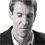Last reply by: Dr. William MurrayFri Dec 15, 2017 12:31 PMPost by Chris DesRochers on December 11, 2017The links to the additional problems are not working. They link to the same lecture problems and not any additional problems. :(Thanks for your help!1 answerLast reply by: Dr. William MurrayThu Nov 20, 2014 3:20 PMPost by David Llewellyn on November 19, 2014How do the table and the graph in the answer to the practice question relate to the actual question? Shouldn't the graph show the relationship between x and y and not the two relationships between x (albeit a different function than in the question) and y and t?1 answerLast reply by: Dr. William MurrayFri Aug 16, 2013 6:21 PMPost by Timothy Davis on August 14, 2013Hi Dr. Murray,   Thanks for answering my last question.  I thoroughly enjoy your videos.   You mentioned that once we find the tangent line, we can use the point slope formula to find the equation of the tangent line.  My question is, if we have the slope of the tangent line already defined by dy/dt / dx /dt, why do we need to find the equation of the tangent line?  What is the difference between the slope of the tangent line and the equation of the tangent line?  You go over this around 1:15 into your lecture.1 answerLast reply by: Dr. William MurrayWed Apr 3, 2013 11:52 AMPost by omatseye ugen on March 12, 2012good job . but i thought this video will involve mostly multivar. calculus1 answerLast reply by: Dr. William MurrayWed Apr 3, 2013 11:48 AMPost by Allison Walsh on April 6, 2011Awesome!6 answersLast reply by: Dr. William MurrayWed Apr 3, 2013 11:46 AMPost by Wen Geng on March 7, 2011Dr. Murray's lectures are clear, quick and concise. Very good, unlike the Chemistry ones.

### Parametric Curves

Main formula:

Slope m =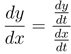Arclength =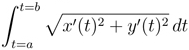Hints and tips:

• You can remember the formula for the slope of the tangent line by thinking that symbolically, the dt’s cancel, leaving you with dydx.

• To find the equation of the tangent line, you also need a point. Use the given value of t into x(t) and y(t) to find it. Then you can use the point-slope formula from high school algebra (y − y0 = m(x − x0 )) to find the equation.

• Sometimes you aren’t given a value of t, but the coordinates (x, y) instead. Then you must find which value of t gives you the correct (x(t), y(t)). Make sure you check that both x and y are correct for your value of t.

• You can remember the arclength formula by recalling that it is derived from the distance formula between two points, which in turn comes from the Pythagorean Theorem.

• Don’t make the common algebraic mistake of thinking that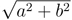reduces to a + b! This is extremely wrong, and your teacher will likely be merciless if you do it

• Many problems in Calculus II classes are “rigged” so that when you expand x′(t)² + y′(t , it becomes a perfect square that cancels nicely with the square root.

• Often this perfect square is achieved by making one of x′(t and y′(t be something of the form (a − b)² = a² − 2ab + b². Then the other one changes it to a² + 2ab + b², which you can then factor as (a + b.

• Another common technique in arclength problems is to make a u-substitution for whatever is under the square root sign. Then (hopefully) you can manipulate the expression outside the square root into being the du. However, you might have to do several steps of algebraic manipulation, pulling factors in or out of the square root sign, before this works.

• You may sometimes be able to use symmetry to find the arclength of part of a curve and then multiply by an appropriate factor to get the total arclength. This can be especially helpful if you just want to examine part of the curve where all the quantities involved are positive.

• When it’s feasible, check that your answer makes sense. Unlike area integrals, which can be negative if a curve goes below the x-axis, arclength should always be positive! You might also be able to check that the curve looks about as long as your answer.

### Parametric Curves

Graph f(t) = (sin(x), √x) for 0 ≤ t ≤ 2π
• Make a table
•  t
 0
 2
 π
 [(3π)/2]
 2π
 x(t)
 0
 1
 0
 − 1
 0
 y(t)
 0
 1.25
 1.77
 2.17
 2.51
Plot the points and graph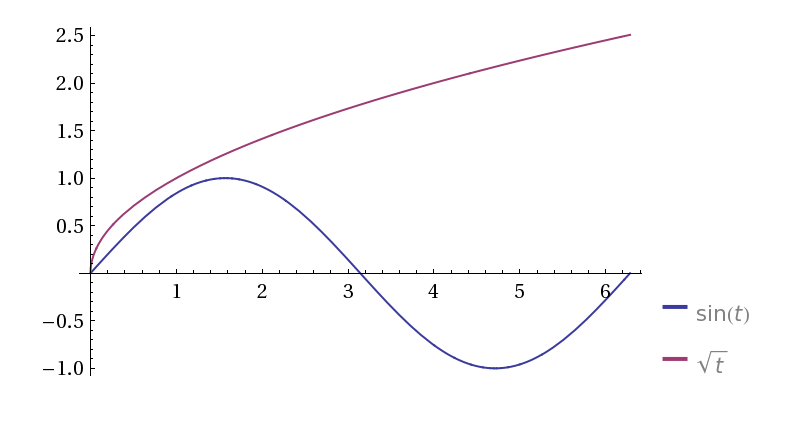A parametric curve is defined by
y(t) = t2
x(t) = t + 2
What is its Cartesian equation?
• Solve for t with x
• x = t + 2
• t = x − 2
Substitute into y(t)
y(t) = t2
y = (x − 2)2
A parametric curve is defined by:
y(t) = t − 9
x(t) = √t
for t ≥ 0
What is its Cartesian equation?
• x = √t
• t = x2
Substitute into y(t)
y(t) = t − 9
y = x2 − 9
Find the Cartesian equation of the following parametric equations:
x(t) = [cos(t)/3]
y(t) = [sin(t)/4]
for t ≥ 0
• Isolate x and y
• x = [cos(t)/3]
• 3x = cos(t)
• y = [sin(t)/4]
• 4y = sin(t)
Apply Pythagorean identity
cos2(t) + sin2(t) = 1
(3x)2 + (4y)2 = 1
9x2 + 16y2 = 1
Find the Cartesian equation of the following parametric equations:
x(t) = √2 sin(t)
y(t) = 2cos(t) + 3
for t ≥ 0
• Isolate x
• x = √2 sin(t)
• [x/(√2 )] = sin(t)
• Isolate y
• y = 2cos(t) + 3
• y − 3 = 2cos(t)
• [(y − 3)/2] = cos(t)
sin2(t) + cos2(t) = 1
([x/(√2 )])2 + ([(y − 3)/2])2 = 1
[(x2)/2] + [((y − 3)2)/4] = 1
Find the Cartesian equation of the following parametric equations:
x(t) = t + 5
y(t) = t3 + 5
• Isolate t with x
• x = t + 5
• x − 5 = t
y = (x − 5)3 + 125
y = x3 − 15x2 + 75x − 125 + 125
y = x3 − 15x2 + 75x
Find the Cartesian equation of the following parametric equations:
x(t) = 1 + t
y(t) = t2 − 9
• Isolate t with x
• x = 1 + t
• − t = 1 − x
• t = x − 1
Substitute into y(t)
y = (x − 1)2 − 9
y = x2 − 2x + 1 − 9
y = x2 − 2x − 8
Find the Cartesian equation of the following parametric equations, and graph it:
x(t) = t2 − 3
y(t) = 1 − t
for t − 3
• Isolate t with x
• x = t2 − 3
• x + 3 = t2
• √{x + 3} = t
• Substitute into y(t)
• y = 1 − √{x + 3}
• Graph the equation using shifts
• y = 1 − √{x + 3}
The graph √x has been shifted up by 1 unit to y = 1, and left by 3 units. It also has been reflected.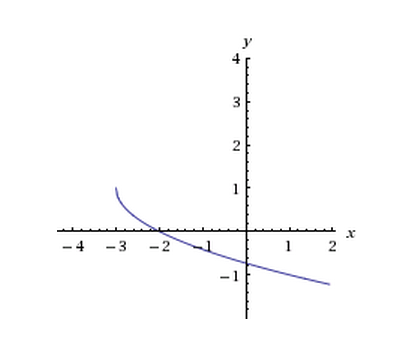Find the Cartesian equation of the following parametric equations, and graph it:
x(t) = arccos([t/4]) − [(π)/2]
y(t) = [t/8] − 3 for − 2π ≤ t ≤ 2π
• Isolate t with x
• x = arccos([t/4]) − [(π)/2]
• x + [(π)/2] = arccos([t/4])
• cos(x + [(π)/2]) = [t/4]
• 4cos(x + [(π)/2]) = t
• Observe half - angle trig identity
• cos(u + [(π)/2]) = − sinu
• 4cos(x + [(π)/2]) = t
• − 4sinx = t
• Substitute into y(t)
• y = [( − 4sin(x))/8] − 3
• y = − [sinx/2] − 3
• Graph using shifts, reflections, and stretching
• y = − [sinx/2] − 3
• The graph y = sinx has been shifted 3 units down to y = − 3, and reflected. It also has been compressed by a factor of 2.
• Remember to graph within the bounds − 2π ≤ t ≤ 2π.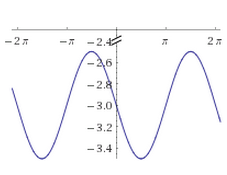Find the length of the arc of y = (x − 8)[3/2] from − 10 to 17
• Find y′
• y′ = [3/2](x − 8)1/2dx
• Use Arc Length Formula
• Arc Length = ∫ab√{1 + ( [dy/dx] )2} dx
• = ∫1017 √{1 + ( [3/2](x − 8)1/2 )2} d x
• = ∫1017 √{1 + ( [9/4](x − 8) )} dx
• = ∫1017√{1 + [9/4]x − 18} dx
• = ∫1017 √{[9/4]x − 17} dx
• Use u substitution
• u = [9/4]x − 17
• du = [9/4]dx
• 1017 √{[9/4]x − 17} dx = ∫1017 [4/9]√u du
• = [4/9]∫1017 √u du
• = [4/9][ [2/3]u[3/2] ]1017
• = [4/9][ [2/3]( [9/4]x − 17 )[3/2] ]1017
≈ 25.20
Find the derivative of − lncosx
• Use log properties and trig identities to alter
• − lncosx = ln( cosx ) − 1
• = ln[1/cosx]
• = lnsecx
Use chain rule
[dy/dx] = [secxtanx/secx] = tanx dx
From 1 to 3, find the length of y = − lncosx
• Find the derivative of − lncosx
• y′ = tanx
• Apply Arc Length equation
• ab√{1 + ( [dy/dx] )2} dx = ∫13√{1 + ( tanx )2} dx
• = ∫13√{1 + tan2x} dx
• Use Pythagorean identity
• 13√{1 + tan2x} dx = ∫13√{sec2x} dx
• = ∫13 secxdx
• Use trig integral identity
• secxdx = ln|secx + tanx| + C
• 13 secxdx = [ ln|secx + tanx| ]13
• = ln|sec(3) + tan(3)| − ln|sec(1) + tan(1)|
≈ − 1.08
Find the length of − 1 to 4 of the follow parametric functions:
x = [2/3]t[3/2], y = 2t + 7
• Find the deratives using product rule
• x′ = √t
• y′ = 2
• Apply the parametric Arc Length Formula
• Arc Length = ∫ab √{( [dx/dt] )2 + ( [dy/dt] )2} dt
• = ∫ − 14 √{( √t )2 + ( 2 )2} dt
• = ∫ − 14 √{t + 4} dt
• Use substitution with u = t + 4
• du = dt
• − 14 √{t + 4} dt = ∫ − 14 √u du
• = [3/2][ t + 4 ] − 14
• = [3/2]( 4 + 4 − ( − 1 + 4) )
• = [3/2]( 8 + 3 )
= [33/2]
Find the length of 0 to 3 of the follow parametric functions:
• x = etcost, y = etsint
• Find the deratives using product rule
• x′ = etcost − etsint
• y′ = etsint + etcost
• Apply the parametric Arc Length Formula
• Arc Length = ∫ab √{( [dx/dt] )2 + ( [dy/dt] )2} dt
• = ∫03 √{( etcost − etsint )2 + ( etsint + etcost )2} dt
• = ∫03 √{2e2t(cos2t + sin2t)} dt
• = ∫03 √{2e2t} dt
• = √2 ∫03 etdt
• = √2 [ et ]03
= √2 [ e3 − e0 ] = √2 [ e3 − 1 ]
Find the length of 1 to [(3π)/4] of the follow parametric functions:
• x = ln|sint|, y = t
• Find the deratives using trig integral identity
• x′ = cott
• y′ = 1
• Apply the parametric Arc Length Formula
• Arc Length = ∫ab √{( [dx/dt] )2 + ( [dy/dt] )2} dt
• = ∫1[(3π)/4] √{( cott )2 + ( 1 )2} dt
• = ∫1[(3π)/4] √{cot2t + 1} dt
• Use Pythagoream Identity
• 1[(3π)/4] √{cot2x + 1} dt = ∫1[(3π)/4] √{csc2x} dt
• = ∫1[(3π)/4] csctdt
• = [ − ln|csct + cott| ]1[(3π)/4]
≈ 1.49
Find the arc length of the polar function r = 2cosθ from 0 to 5π
• Find [dr/(dθ)]
• r′ = − 2sinθ
• Apply the polar Arc Length Formula
• Arc Length = ∫ab √{r2 + ( [dr/(dθ)] )2} dθ
• = ∫0 √{( 2cosθ )2 + ( − 2sinθ )2} dθ
• = ∫0 √{4cos2θ+ 4sin2θ} dθ
• = ∫0 √4 √{cos2θ+ sin2θ} dθ
• = 2∫0 √{cos2θ+ sin2θ} dθ
• = 2∫0 √1 dθ
• = 2[ θ]0
= 10π
What is the parametric Arc Length equation of r = cosθ?
• Convert using Polar definitions
• x = rcosθ
• = cosθ(cosθ)
• = cos2θ
• y = rsinθ
• = cosθsin θ
• Find [dx/(dθ)] and [dy/(dθ)]
• x′ = − 2sinθcosθ
• y′ = cos( 2x )
• Apply parametric Arc Length Formula (but not necessarily solve)
• Arc Length = ∫ab √{( [dx/dt] )2 + ( [dy/dt] )2} dt
• = ∫ab √{( [dx/(dθ)] )2 + ( [dy/(dθ)] )2} dθ
= ∫ab √{( − 2sinθcosθ )2 + ( cos( 2x ) )2} dθ
What is the polar Arc Length Equation of r = 2e
• Find [dr/(dθ)]
• r′ = e
• Arc Length = ∫ab √{r2 + ( [dr/(dθ)] )2} dθ
= ∫ab √{4e2 + e} dθ = ∫ab √{5e} dθ = √5 ∫ab e
What's the Arc Length for r = e from 0 to 1?
• Apply previous equation with known bounds
• √5 ∫01 edθ = [(√5 )/2][ e ]01
= [(√5 )/2](e2 − 1)

*These practice questions are only helpful when you work on them offline on a piece of paper and then use the solution steps function to check your answer.

### Parametric Curves

Lecture Slides are screen-captured images of important points in the lecture. Students can download and print out these lecture slide images to do practice problems as well as take notes while watching the lecture.

• Intro 0:00
• Important Equations 0:05
• Slope of Tangent Line
• Arc length
• Lecture Example 1 1:40
• Lecture Example 2 4:23
• Lecture Example 3 8:38

### Transcription: Parametric Curves

Welcome back and we are trying some more examples of arc lengths.0000

And other problems involving parametric equations.0005

We have here the length of a curve given by x(t) = cos2(t) and y(t) = sin2(t) as t goes from 0 to π/2.0010

Remember our arc length formula is x'2 + y'2, take the square root of that and integrate it.0023

Let us calculate x'.0029

X is cos2(t), so that would be 2 × cos(t) × -sin(t) using the chain rule there,0034

The - sign comes from the derivative of cosine.0044

y'(t) is 2 × sin(t) × derivative of sin which is cos(t).0049

If we square each one of those, x'(t)2 is 4cos2(t) sin2(t).0059

y'(t)2 is 4sin2(t) cos2(t).0070

If we add those up, x'2 + y'2,0082

Well those are the same thing.0091

We just get the sqrt(8sin2(t)cos2(t)).0094

The sqrt(8) is 2×sqrt(2).0102

The square root of sin2 and cos2, since sin and cos are positive when t is between 0 and π/2,0105

This is sin(t) × cos(t).0117

So, that is what we want to integrate.0122

The integral from t=0, to t=π/2.0125

I will write the 2×sqrt(2) on the outside.0130

sin(t) cos(t) dt.0138

This integral is not too bad.0143

We can use the substitution u = sin(t).0145

The reason that works so well is because du = cos(t) dt.0149

So, what we have here is the integral of u du.0156

That gives us u2/2.0160

Now to convert things back into t.0169

2×sqrt(2) × sin2(t)/2.0172

Evaluated from t=0 to t=π/2.0180

Those 2's cancel each other so we get sqrt(2) × sin(π/2)2,0188

That is just one.0195

Minus sin(0)2, which is just 0.0197

So our arc length is just sqrt(2).0200

Again there, the calculus worked nicely.0206

What we did was find x' and y' and plugged them into this Pythagorean Formula.0208

Then we integrated to get the answer.0215

There is actually another way to see how this problem works.0218

We can see through this problem geometrically.0220

If you actually try graph the path that these equations are describing,0226

Notice that cos2(t) + sin2(t) = 1.0228

So, cos2(t) = x, sin2(t) = y.0240

So this path is actually taking place on the line y+x = 1.0245

So there is that line,0255

And if you plug in t=0, then x=1 and y=0.0257

There is t=0.0264

At the point (1,0).0266

If you plug in t - π/2, then y = 1 and x=0.0269

So, there is t=π/2 at the point (0,1).0277

So, what this path is really doing is just following a straight line from (1,0) to (0,1).0286

Of course the length of that line is sqrt(2).0293

That is kind of a geometric check on the calculus we just did.0297

Let us try one more example.0000

We want to find the length of the curve given by x(t) = 7 + 2t,0002

And y(t) = et + e-t, where t goes from 0 to 1.0006

Again we want to find x' and y', square each one, add them up and take the square root.0013

x'(t) = 2, y'(t), y = et + e-t, so the derivative of et is just et.0020

The derivative of e-t is e-t × the derivative of -t, which is -1.0037

So we get -e-t.0047

So x'(t)2 + y'(t)2.0050

Well, 22 is just 4.0058

Now, if we square y'(t), then we are going to follow the formula (a + b)2,0060

Is a2 + 2ab + b2.0074

Here a is et, so (et)2 is e2t, b is -e-t,0080

So this is -2ab, well ab is et × e-t,0090

Then + b2 is e-t, so that is e-2t,0102

But look at this et × e-t, is e0 which is 1.0111

This is just -2.0116

We also have a 4 here, so what we get is e2t - 2 + 4, is just + 2.0120

+ e-2t.0131

The clever thing to do here is to write that 2 as 2et × e-t again + e-2t.0134

This is (et + e-t)2.0145

The square root of all that, x'(t)2 + y'(t)2.0155

The square root cancels that for a fixed square,0164

So we get et + e-t.0168

That is what we want to integrate to find the arc length.0173

We take the integral from t=0 to t=1.0175

We are getting those bounds from the original problem of et + e-t dt.0181

That integral is not too bad.0192

The integral of et, is just et itself.0194

The integral of e-t, is e-t/the derivative of -t, which is -1.0197

That is the same as multiplying by -1.0207

So this is et - e-t.0210

Then we want to evaluate that from t=0 to t=1.0213

That is e1 - e-1 - e0 + e0.0221

The e0's are both 1 so those cancel each other out.0232

This is e - e-1.0235

I will write this as 1/e, and that is our answer for the arc length.0240

Again, what we did there, we looked at the x and y that we were given,0246

We took the derivative of each one, squared them, added them up, took their square root.0252

And integrated to get our answer for the arc length.0257

Thanks for watching, this has been educator.com.0261

Hi this is educator.com and we are here to talk about parametric curves.0000

The idea about parametric curves is that you are given the equation x(t) and y(t) and those define how a point is moving around in the plane.0007

The x(t) gives you the x coordinate at a particular time and the y(t) gives you the y coordinate at a particular time.0019

There are basically two calculus problems associated with parametric equations.0026

One is to find the tangent line to occur at a particular point.0030

The way you are going to do that is we will fine the slope of the tangent line by looking at d(y) dt.0039

So, just looking at the derivative of the y equation, and dividing by d(x) d(t), which is the derivative of the x equation.0048

That will give us the slope of the tangent line and we will also know one point on the tangent line.0052

We can use the point slope equation to find the slope of the tangent line.0059

The other equation that you use with parametric equations a lot is0063

You find the length of a curve and essentially the formula from that comes from the Pythagorean distance formula.0075

You look at x'(t2 + y'(t2) and then you find the square root of that.0076

That is a unit of arc length representing the length travelled in a very small amount of time.0084

Then you integrate that from your starting time to your ending time and that formula represents the total length of the curve.0089

Let us try this out with some examples0097

The first examples is the equations are x(t) = t+1, y(t) = t2.0100

Maybe I will just graph a couple of points there.0109

If t = 0 then x = 1 and y = 0.0111

If t = 1 then x = 2 and y = 1.0118

If t = 2 then x = 3 and y = 4.0125

This point is travelling along a parabolic path here.0133

What we are asked to do is find the tangent line at t=1.0138

At t=1, remember, x = 2 and y = 1.0143

We are trying to find the tangent line at that point right there.0149

We need to find the slope but our slope is d(y) dt/d(x) dt.0156

Now dy dt, since y = t2 is 2t.0170

Dx dt, since x = t+1 is just 1.0174

That is 2t, and when we plug in t = 1, that gives us the slope of 2.0180

Now we have the slope and we have a point and it is just an algebra problem to find the equation of a line.0189

We use the point slope formula, y - y0, which is 1 here, is equal to the slope × x - x0 which is 2.0195

This is 2x - 4.0207

We get the equation of the tangent line, y = 2x-3.0213

To recap there, what we are trying to do is to find the tangent line to a curve that is defined by parametric equations.0220

What we did was we plugged in the time value we were given, t =1 to get a point on the tangent line.0230

Then we used our equation for the slope dy dt/dx dt.0240

We figured those out using the equations for x and y that we were given.0245

We plugged in the same value of t and we got our slope and then we had a point on our slope,0250

And we could use the old point-slope formula to find the equation for our tangent line.0259

Let us find another tangent line.0263

This time the curve is x(t) = cos(t) y(t) = sin(t).0265

You will hopefully recognize that as the equations for a circle because cos2(t) + sin2(t) = 1.0272

Those are the equations that define a point moving around in a circle.0282

We want to find the tangent line at the point, (sqrt(3/2), 1/2).0288

That is about right there on the circle0294

The difference between this one and the previous one is we have not been given a t value.0297

We have to figure out what value of t gives us the point (sqrt(3/2), 1/2).0302

What value of t if you plug it in to cos(t) sin(t) gives us the sqrt(3/2)?0308

The answer is pi/6 because the cos(pi/6) is sqrt(3/2) and the sin(pi/6) is 1/2.0318

So, we know that t is pi/6.0328

Again, to find the slope, we use dy dt/dx dt.0330

Now y = sin(t) so the derivative there is cos(t).0340

X = cos(t), the derivative of that is -sin(t).0345

Then we plug in the value t = pi/6 to get a number for the slope so the cos(pi/6) = sqrt(3/2)0354

The sin(pi/6) = 1/2, and we still have our negative there.0364

The two's cancel so we get our slope is -sqrt(3).0370

That gives us the slope and we also have a point, so I will plug those into the point slope formula.0376

y - 1/2 = -sqrt(3) x - sqrt(3/2).0380

Those values are coming from plugging the points in there.0394

We can simplify this a bit.0396

This is = sqrt(3)x.0398

The minuses cancel each other out plus sqrt(3) × sqrt(3) = 3.0400

Then we can bring this half over to the other side so we get y = -sqrt(3)x + 3/2 + 1/2, is just 2.0410

We get our tangent line to be y = -sqrt(3)x + 2.0429

The way that this problem was different from the previous one was that we were not given a value of t.0435

We had to look at the point we were given and we had to figure out what the value of t should be.0440

From then on we used the same formulas to find out the slope and the equation of the tangent line.0450

By the way, this problem you can also check the answer geometrically if you draw that tangent line.0454

It forms a 30-60-90 triangle with the x and y axis.0464

That is 30 degrees and that is 60 degrees.0470

We know what those angles are because the tangent line is perpendicular to the radius of the circle there,0476

We also know that the radius of the circle is 1 so we have a smaller 30-60-90 triangle in there.0484

With a short side of length 1, so the long side has length 2.0501

That confirms that the y intercept of this tangent line = 2.0506

That is a little check on our work using some trigonometry and no calculus at all0511

Let us try an example of arc length now.0519

We are given the curve x(t) = 6 - 2t3, y(t) = 8 + 3t2.0520

We want to find the length of that curve from t = 0 to t = 2.0530

Remember our arc length formula says you want to take the integral of sqrt of x'(t)2 + y'(t)2 dt.0536

x'(t), the derivative of x if x is 6 - 2t3,0549

You take its derivative and the 6 just goes away, so the derivative is -6t2.0556

y'(t), if y' is 8 + 3t2, again the 8 does not have any effect.0568

The 3t2 you take the derivative and you get 6t.0575

What we want then is sqrt(x')2, well that is (-6t)2, so that is 36t40584

+ y'2 is 36t2.0596

That can simplify because we can pull a 36 out and just get 6,0600

we can pull a t2 out and get t.0610

Under the radical, what we have left is just t2 + 1.0615

The arc length = integral from t=0 to t=2 of 6t × sqrt(t2+1) dt.0620

Now, this integral is not too bad because what we can do is make a little substitution.0645

u = t2 + 1.0650

Then, du = 2t, dt.0654

The reason that works so nicely is that we already have the t and the dt, so we basically have du.0658

In fact, 6t dt, is just 3 du.0666

Then, we still have the integral from t = 0 to t = 2 of now the sqrt(u) du.0674

You can think of the square root as u1/2.0683

To integrate that we get u3/2/3/2 which is the same as multiplying by 2/3.0687

Then we still have that 3 on the outside.0698

This is evaluated from t=0 to t=2.0701

I have to convert the u's back into t's0706

These 3's cancel, so we get 2 × u was (t2 + 1)3/2 evaluated from t=0 to t=2.0709

This gives us 2 now if we plug in t=2, we get 22 + 1, so that is 53/2.0727

- 02 + 1, so that is 13/2.0737

We can simplify that to a little bit.0744

53/2 is the same as 5 × 51/2.0746

13/2 is just 1.0749

We get our final answer for the arc length there.0758

What made this problem work is having this formula that came from the pythagorean theorem.0762

We just take the x(t) and the y(t) that we are given and we plug them into this formula which involves computing a couple derivatives,0768

Simplifying a little bit if we can, then we integrate and we get out answer.0775

We will try a couple more examples later.0782

OR

### Start Learning Now

Our free lessons will get you started (Adobe Flash® required).